# Search by Topic

Filter by: Content type:
Age range:
Challenge level:

### There are 21 results

Broad Topics > Numbers and the Number System > Representing numbers### Eightness of Eight

##### Age 5 to 7 Challenge Level:

What do you see as you watch this video? Can you create a similar video for the number 12?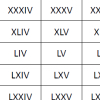### Roman Numerals

##### Age 7 to 11 Challenge Level:

Can you find some examples when the number of Roman numerals is fewer than the number of Arabic numerals for the same number?### Dotty Six

##### Age 5 to 11 Challenge Level:

Dotty Six is a simple dice game that you can adapt in many ways.### Even and Odd

##### Age 5 to 7 Challenge Level:

This activity is best done with a whole class or in a large group. Can you match the cards? What happens when you add pairs of the numbers together?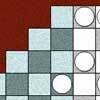### Napier's Location Arithmetic

##### Age 14 to 16 Challenge Level:

Have you seen this way of doing multiplication ?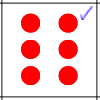### Dotty Six for Two

##### Age 5 to 11 Challenge Level:

Dotty Six game for an adult and child. Will you be the first to have three sixes in a straight line?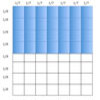### Models in Mind

##### Age 3 to 14

This article looks at how models support mathematical thinking about numbers and the number system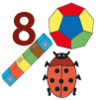### Matching Numbers

##### Age 5 to 7 Challenge Level:

Can you find different ways of showing the same number? Try this matching game and see!### Galley Division

##### Age 14 to 16 Challenge Level:

Can you explain how Galley Division works?### Balance Power

##### Age 11 to 18 Challenge Level:

Using balancing scales what is the least number of weights needed to weigh all integer masses from 1 to 1000? Placing some of the weights in the same pan as the object how many are needed?### Twizzle Twists

##### Age 14 to 16 Challenge Level:

Make the twizzle twist on its spot and so work out the hidden link.### A Story about Absolutely Nothing

##### Age 7 to 18

This article for the young and old talks about the origins of our number system and the important role zero has to play in it.### Twizzle Wind Up

##### Age 14 to 16 Challenge Level:

A loopy exploration of z^2+1=0 (z squared plus one) with an eye on winding numbers. Try not to get dizzy!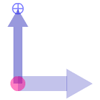### Twizzle Arithmetic

##### Age 14 to 16 Challenge Level:

Arrow arithmetic, but with a twist.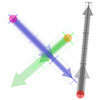### Arrow Arithmetic 3

##### Age 14 to 16 Challenge Level:

How can you use twizzles to multiply and divide?### Arrow Arithmetic 2

##### Age 14 to 16 Challenge Level:

Introduces the idea of a twizzle to represent number and asks how one can use this representation to add and subtract geometrically.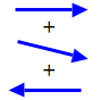### Arrow Arithmetic 1

##### Age 14 to 16 Challenge Level:

The first part of an investigation into how to represent numbers using geometric transformations that ultimately leads us to discover numbers not on the number line.### Multiplication Series: Illustrating Number Properties with Arrays

##### Age 5 to 11

This article for teachers describes how modelling number properties involving multiplication using an array of objects not only allows children to represent their thinking with concrete materials,. . . .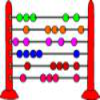### Fibonacci's Three Wishes 1

##### Age 7 to 14

First or two articles about Fibonacci, written for students.### Multiplication Series: Number Arrays

##### Age 5 to 11

This article for teachers describes how number arrays can be a useful reprentation for many number concepts.### A Romantic Riddle

##### Age 7 to 11 Challenge Level:

Read this riddle and see if you can work out how the trees must be planted.